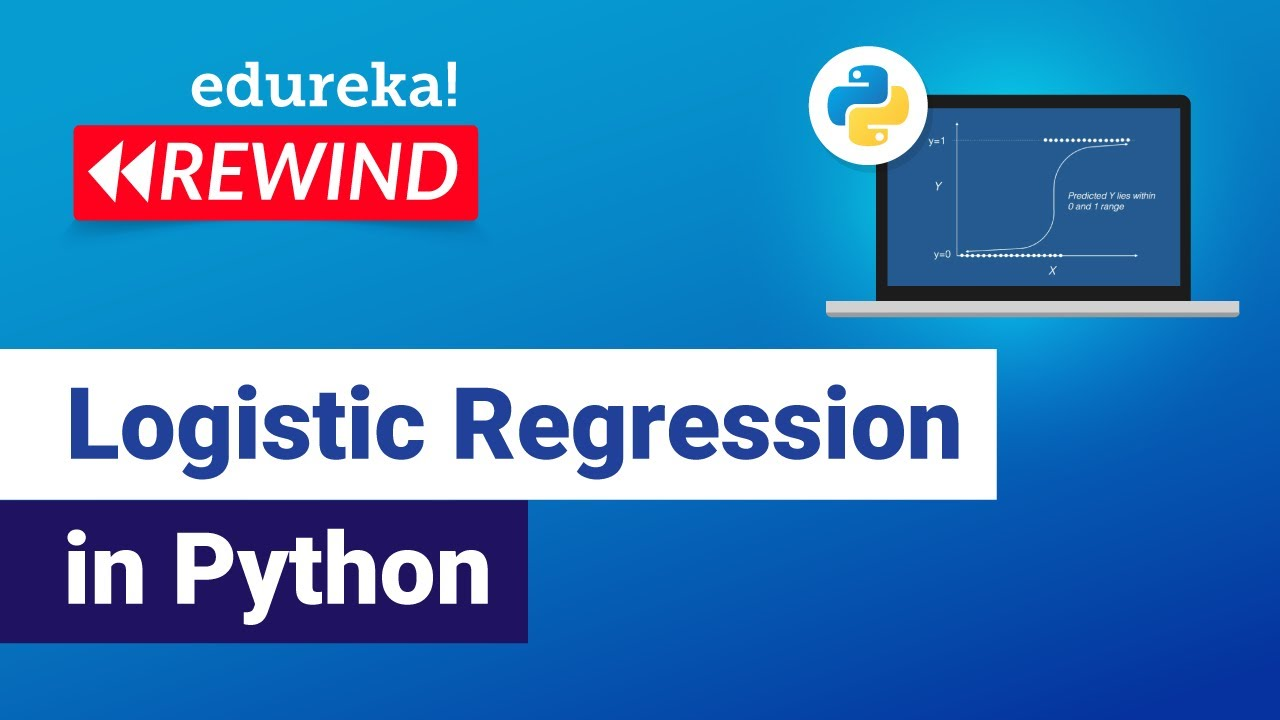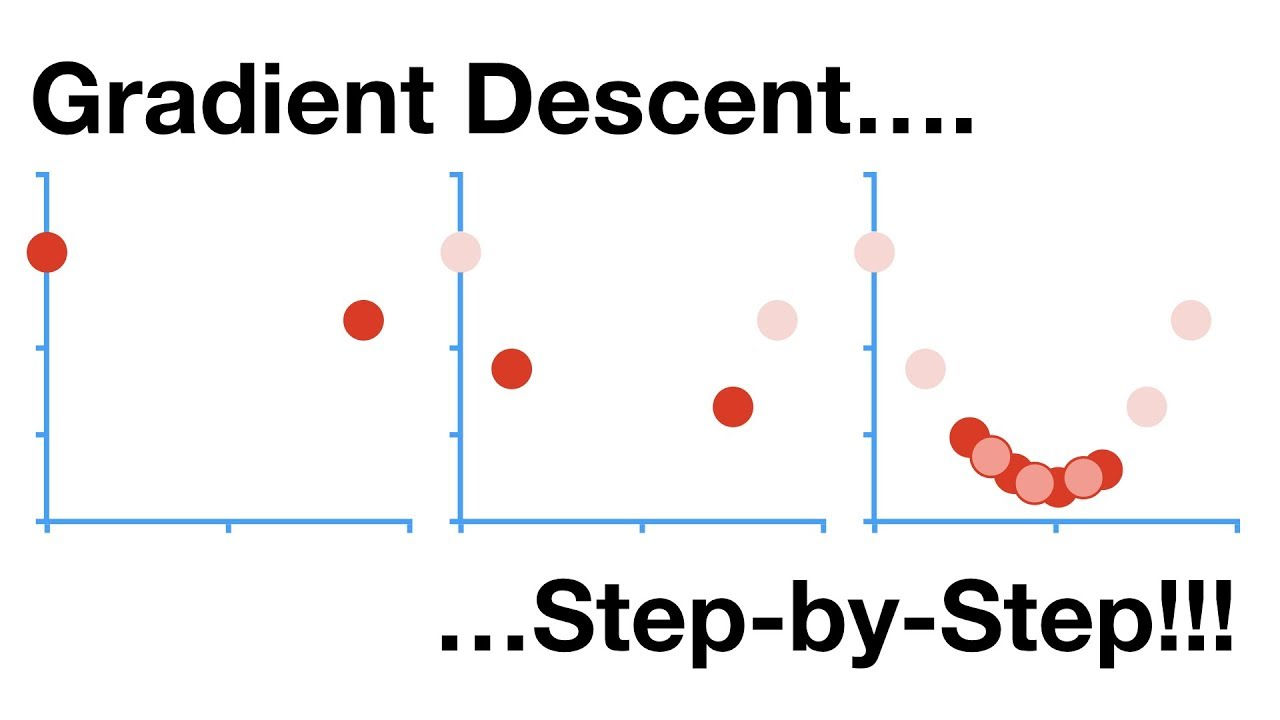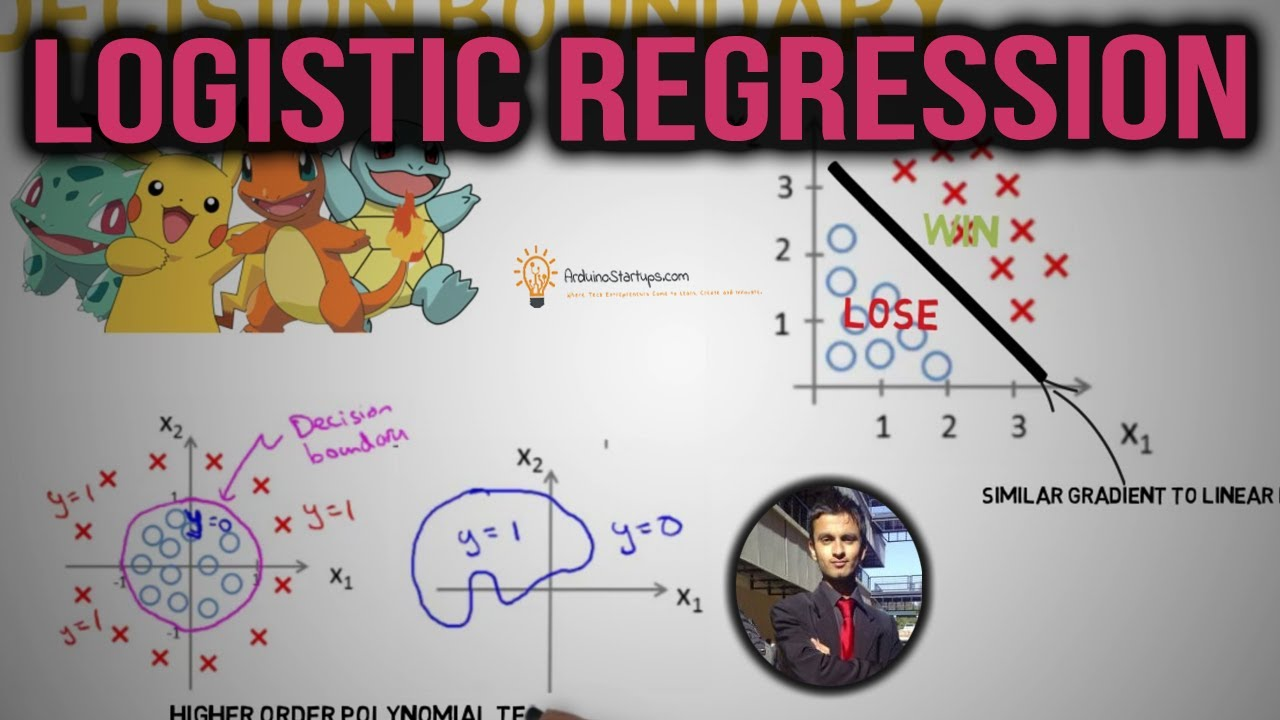# Logistic RegressionPython

#### Logistic Regression in Python

This Edureka Video on Logistic Regression in Python will give you basic understanding of Logistic Regression Machine Learning Algorithm with examples. Time stamps: 00:00 Introduction 00:31 Agenda 01:13 What is Regression 03:25 Logistic Regression- What and Why 04:10 Why not Linear Regression 05:18 Logistic Regression curve 06:57 Logistic Regression Equation 08:49 Linear vs Logistic Regression […]AI Data Science Machine Learning

#### A Step-by-Step Look at Gradient Descent

Gradient Descent is the workhorse behind much of Machine Learning. When you fit a machine learning method to a training dataset, you’re almost certainly using Gradient Descent. The process can optimize parameters in a wide variety of settings. Since it’s so fundamental to Machine Learning, Josh Starmer of StatQuest decided to make a “step-by-step” video […]